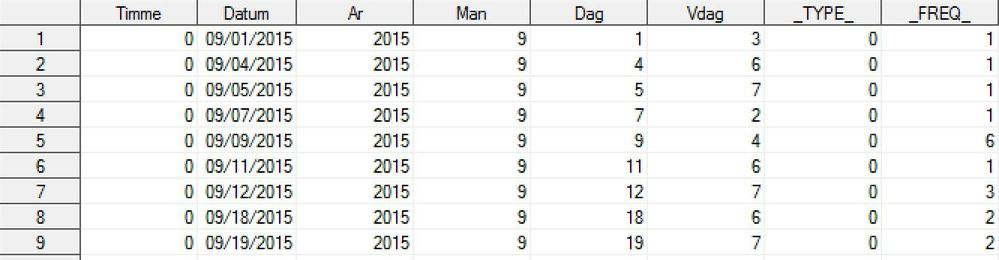## Possible to add a row to dataset for missing values?

Hello,

I'm stuck on a problem here that I hope there is a solution for somewhere in the SAS-commands.

The data looks like this:The raw-data consists of number of cars arriving at a certain location. There is a file when they arrive (this one) and one when they leave. The data of interest is datum (date), timme (hour) and freq ) sum of number of cars arriving during that hour that date.

In the raw-data there might be some hours certain days when no cars arrive, as you can see above (hour 0, 09/02/2015 for example).

I need to match this file with the other to get the total sum of cars present every hour, taking the sum of arriving cars and subtract the leaving.

Problem is I need to have rows even when there are 0 cars arriving, not a missing row like seen above.

Is there any way I can make a loop or something similar to check if there are rows for every hour (0-23), every day (whole 2015). If the row is missing SAS will add the row with the date and hour missing with FREQ=0. Is this possible?

Regards

Peter Karlsson

3 REPLIES 3

## Re: Possible to add a row to dataset for missing values?

Hi,

Lets start with the usual, post test data in the form of a datastep, a picture is useless to us.  Also show what output you need.  From what you post, I cannot see a row for 09/02/2015, nor any logic why that should be added and not 09/03/2017?

To answer you final question, yes loops are one method:

```data want;
set have;
retain lstdt;
if _n_=1 then lstdt=start_date;
else do;
if start_date + 1 ne lstdt then do;
do i=start_date to lstdt;
output;
start_date=start_date+i;
end;
end;
else output;
end;
run;
```

Something like that.

## Re: Possible to add a row to dataset for missing values?

You can make a dummy data set of DATUM and TIMME values for 2015. Then interleave with datasets ARRIVE and LEAVE:

``````data date_time_dummies;
do datum='01jan2015'd to '31dec2015'd;
do timme=0 to 23;
output;
end;
end;
format datum mmddyy10.;
run;

data want (keep=datum timme total);
set arrive (in=ina) leave (in=inL) date_time_dummies;
by datum timme;
retain total 0;

if ina then total=total+_freq_;
else if inL then total=total-_freq_;
if last.timme;
run;
``````
--------------------------
The hash OUTPUT method will overwrite a SAS data set, but not append. That can be costly. Consider voting for Add a HASH object method which would append a hash object to an existing SAS data set

Would enabling PROC SORT to simultaneously output multiple datasets be useful? Then vote for
Allow PROC SORT to output multiple datasets

--------------------------

## Re: Possible to add a row to dataset for missing values?

Hi!

I'm sorry for not getting back to you before. I have been away for some days but now I'm back.

We have decided to go with another solution so this case can be closed 🙂

thanks for the input though!

Regards

Peter Karlsson

Discussion stats
• 3 replies
• 2275 views
• 0 likes
• 3 in conversation